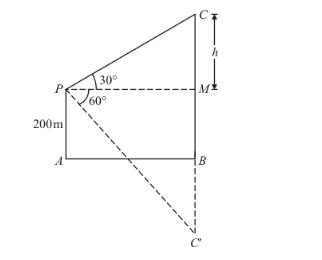# If the angle of elevation of a cloud from a point 200 m above a lake is 30°

Question:

If the angle of elevation of a cloud from a point 200 m above a lake is 30° and the angle of depression of its reflection in the lake is 60°, then the height of the cloud above the lake is

(a) 200 m

(b) 500 m

(c) 30 m

(d) 400 m

Solution:

Letbe the surface of the lake andbe the point of observation. Som.

The given situation can be represented as,Here,is the position of the cloud andis the reflection in the lake. Then.

Let $P M$ be the perpendicular from $P$ on $C B$. Then $\angle C P M=30^{\circ}$ and $\angle C^{\top} P M=60^{\circ}$.

Let $C M=h, P M=x$, then $C B=h+200$ and $C^{\prime} B=h+200$

Here, we have to find the height of cloud.

So we use trigonometric ratios.

In,

$\Rightarrow \tan 30^{\circ}=\frac{C M}{P M}$

$\Rightarrow \frac{1}{\sqrt{3}}=\frac{h}{x}$

$\Rightarrow x=\sqrt{3} h$

Again in $\triangle P M C^{\prime}$,

$\Rightarrow \tan 60^{\prime}=\frac{C^{\prime} M}{P M}$

$\Rightarrow \sqrt{3}=\frac{C^{\prime} B+B M}{P M}$

$\Rightarrow \sqrt{3}=\frac{h+200+200}{x}$

$\Rightarrow \sqrt{3} x=h+400$

Put $x=\sqrt{3} h$

$\Rightarrow 3 h=h+400$

$\Rightarrow 2 h=400$

$\Rightarrow h=200$

Now,

$\Rightarrow C B=h+200$

$\Rightarrow C B=200+200$

$\Rightarrow C B=400$

Hence the correct answer is d.